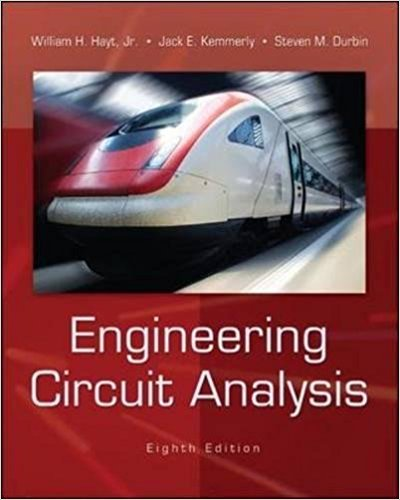×
×

# Find f (t) at t = 2 if F(j) is equal to (a) 5e j3 j(4/); (b) 8[( 3) + ( + 3)]; (c)ISBN: 9780073529578 304

## Solution for problem 18.13 Chapter 18.7

Engineering Circuit Analysis | 8th Edition

• Textbook Solutions
• 2901 Step-by-step solutions solved by professors and subject experts
• Get 24/7 help from StudySoup virtual teaching assistantsEngineering Circuit Analysis | 8th Edition

4 5 1 422 Reviews
20
0
Problem 18.13

Find f (t) at t = 2 if F(j) is equal to (a) 5e j3 j(4/); (b) 8[( 3) + ( + 3)]; (c) (8/)sin 5.

Step-by-Step Solution:
Step 1 of 3

Linux O/S -Student Lab-2016: MULTIMEDIAUNIVERSITY Goals of this lab:  Learn the basics of the Linux command line  Learn to manage files and directories  Learn about the command shell  Learn about environment variables  Learn about processes and job control  GROUP MEMBERS 1.Reg. Number: ENG:219-069/2015 Names: LYDIAKIMATA 2.Reg. Number:ENG:219-046/2015...

Step 2 of 3

Step 3 of 3

##### ISBN: 9780073529578

Unlock Textbook Solution